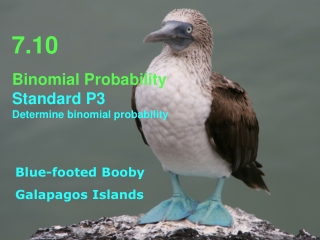DownloadDownload Presentation7.10

# 7.10

Download Presentation## 7.10

- - - - - - - - - - - - - - - - - - - - - - - - - - - E N D - - - - - - - - - - - - - - - - - - - - - - - - - - -
##### Presentation Transcript

1. 7.10 • Binomial Probability • Standard P3 Determine binomial probability Blue-footed Booby Galapagos Islands

2. It’s a binomial problem n=8 and p = .73. P(5) = .2285 The probability that a blue-footed booby will catch a fish on a dive is, let’s say, 0.73. What is the probability that this booby will catch exactly 5 fish on his next 8 dives?

3. P(Fail, Fail, Success)= .27۰.27۰.73 = .0532 The probability that a blue-footed booby will catch a fish on a dive is, let’s say, 0.73. What is the probability that a booby will not catch a fish until her third attempt? (Is this a binomial experiment?)

4. Galapagos tortoises are often found in American zoos. Let’s say the probability a zoo houses at least one Galapageno tortoise is 0.4 and we are studying 75 randomly selected American zoos. What is the probability that not more than 20 of the zoos we are studying have at least one tortoise from the Galapagos? First note the binomial distribution with n = 75 p = .4 and x ≤ 20 using binomcdf P(x ≤ 20) = .0112

5. Why isn’t this a binomial problem? (6/11)(5/10)(4/9)(3/8)(2/7) = .013 If 11 turtles are numbered 1 to 11, what is the probability of randomly choosing a group of 5 turtles with all of them having an odd number? (Is it a binomial experiment?)

6. Probability Ya gotta think! and practice. Try the problems